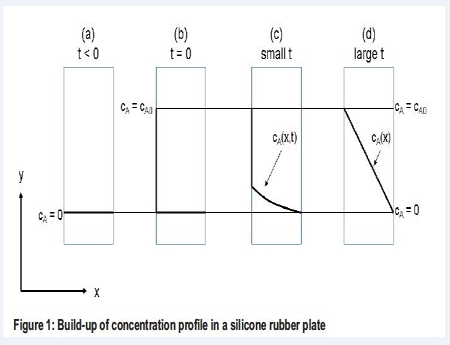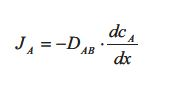# What is Transport of Mass?

Diffusion of mass is also known as Transport of Mass or mass diffusion, concentration diffusion or ordinary diffusion. We are talking about molecular mass transport taking place as diffusion of a component A through a medium consisting of component B. The diffusion coefficient DAB, determines how fast the diffusion takes place. The subscript of the diffusion coefficient tells that the diffusion is associated with the diffusion of A through B. A diffusion situation is sketched in Figure 1 for the diffusion of a gas component A through a plate of silicone rubber.(a) The concentration of A at both sides of the silicone rubber plate is zero. (b) At t = 0 the concentration on the left side of the silicone rubber plate is increased to CAO. (c) Component A starts to diffuse through the silicone rubber. At small values of t, the concentration of A in the silicone rubber is thus a function of both time and distance x. (d) At large values of t, steady state have been established and a linear concentration profile is reached. Thus at steady state the concentration of A is only a function of the distance x in the silicone rubber plate.

(a) The concentration of A at both sides of the silicone rubber plate is zero. (b) At t = 0 the concentration on the left side of the silicone rubber plate is increased to CAO. (c) Component A starts to diffuse through the silicone rubber. At small values of t, the concentration of A in the silicone rubber is thus a function of both time and distance x. (d) At large values of t, steady state have been established and a linear concentration profile is reached. Thus at steady state the concentration of A is only a function of the distance x in the silicone rubber plate.

At steady state the flux of component A through the silicone rubber is given by the flux equation from Table 1, which is called Fick’s law of diffusion:The flux JA is the diffusive flux of component A in the direction x through the silicone rubber plate. The gradient dcA /dx is the concentration gradient of component A inside the rubber plate which is the driving force. DAB is as mentioned earlier the diffusion coefficient of A in B. The value of the diffusion coefficient DAB determines how fast the linear steady state concentration profile develops:

The larger the diffusion coefficient DAB is, the faster the linear steady state concentration profile is reached. If DAB is small, the flux of A is small and the time before steady state is reached is large.

The diffusion coefficient has the units of length2 pr. time:

D, SI – Units : (m2/S)

At constant temperatures and constant low pressures the diffusion coefficient for a binary gas mixture is almost independent of the composition and can thus be considered a constant. It is inversely proportional to the pressure and increases with the temperature. For binary liquid mixtures and for high pressures the behavior of the diffusion coefficient is more complicated and will not be discussed in this text.